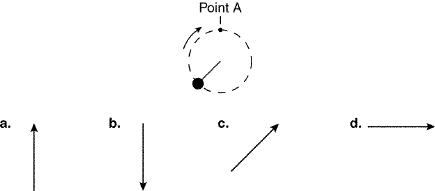Name:     ID:

Email:

Physical Science Chapter 3

True/False
Indicate whether the statement is true or false.

1.

Balanced forces acting on an object cause the object to accelerate.

2.

Gravity causes all falling objects to accelerate at a rate of 98 m/s2.

3.

Acceleration is defined as the rate of change of position.

4.

The momentum of a 5,000-kg truck that is standing still is greater than the momentum of a 3,000-kg truck that is also at rest.

5.

The projectile velocity is the highest velocity that will be reached by a falling object.

6.

When an object falls, it is reacting to the force of gravity.

7.

Jane is on a merry-go-round that is moving at a constant speed. Her velocity is also constant.

8.

Momentum is a property of an object and cannot be transferred from that object to another object.

9.

Objects in Earth's orbit appear to be weightless because they are in free fall.

10.

Friction is a force that encourages motion between two surfaces that are touching each other.

Multiple Choice
Identify the choice that best completes the statement or answers the question.

11.

The upward force on an object falling through the air is ____.
 a. air resistance c. momentum b. inertia d. terminal velocity

12.

The relationship among mass, force, and acceleration is explained by ____.
 a. conservation of momentum c. Newton's second law of motion b. Newton's first law of motion d. Newton's third law of motion

13.

A feather will fall through the air more slowly than a brick because of ____.
 a. air resistance c. inertia b. gravity d. momentum

14.

In the absence of air, a penny and a feather that are dropped from the same height at the same time will ____.
 a. fall at different rates c. float b. fall at the same rate d. not have momentum

15.

The acceleration due to gravity is ____.
 a. 98 m/s2 c. 9.8 m/s b. 9.8 m/s2 d. 0.98 m/s

16.

According to Newton's second law of motion, ____.
 a. F = m ´ a c. F = p ´ a b. F = m ´ v d. F = p ´ v

17.

When an object moves in a circular path, it accelerates toward the center of the circle as a result of ____.
 a. centripetal force c. gravitational force b. frictional force d. momentum

18.

The path of a projectile is ____.
 a. curved c. always vertical b. always horizontal d. straight

19.

For any object, the greater the force that's applied to it, the greater its ____ will be.
 a. acceleration c. inertia b. gravity d. velocity

20.

The size of the gravitational force between two objects depends on their ____.
 a. frictional forces b. inertia c. masses and the distance between them d. speed and direction

21.

As you get farther from the center of Earth, your weight will ____.
 a. decrease c. remain the same b. increase d. can't tell from information given

22.

When a force is exerted on a box, an equal and opposite force is exerted by the box. These forces are called ____ forces.
 a. action-reaction c. frictional b. centripetal d. gravitational

23.

A real car moving at 10 km/h has more momentum than a toy car moving at the same speed because the real car ____.
 a. generates less friction c. has less mass b. has greater mass d. has greater forward motion

24.

In the equation p = m ´ v, the p represents ____.
 a. friction c. momentum b. inertia d. position

25.

The statement "to every action there is an equal and opposite reaction" is ____.
 a. the law of conservation of momentum b. Newton's first law of motion c. Newton's second law of motion d. Newton's third law of motion

26.

The unit of momentum is ____.
 a. kg ´ m c. kg ´ m/s2 b. kg ´ m/s d. m/s2

27.

When two balls collide, the momentum of the balls after the collision is explained by ____.
 a. the law of conservation of momentum b. Newton's first law of motion c. Newton's second law of motion d. Newton's third law of motion

28.

A 300-N force acts on a 25-kg object. The acceleration of the object is ____.
 a. 7,500 m/s2 c. 25 m/s2 b. 300 m/s2 d. 12 m/s2

29.

A 3,000-N force acts on a 200-kg object. The acceleration of the object is ____.
 a. 50 m/s2 c. 15 m/s2 b. 26 m/s2 d. 150 m/s2

30.

An object that is in free fall seems to be ____.
 a. not moving c. speeded up by air resistance b. slowed by air resistance d. weightless

31.

If gravity did NOT affect the path of a horizontally thrown ball, the ball would ____.
 a. go straight up c. follow a curved path b. fall straight down d. travel horizontallyFigure 3-1

32.

A ball attached to a string is being swung in a clockwise circular path as shown in Figure 3-1. Assume the string breaks at point A. In which direction will the ball be traveling an instant later?
 a. direction a c. direction c b. direction b d. direction d

33.

A ball attached to a string is being swung in a clockwise circular path as shown in Figure 3-1. In which direction will the acceleration on the ball be when the ball passes point A?
 a. direction a c. direction c b. direction b d. direction d